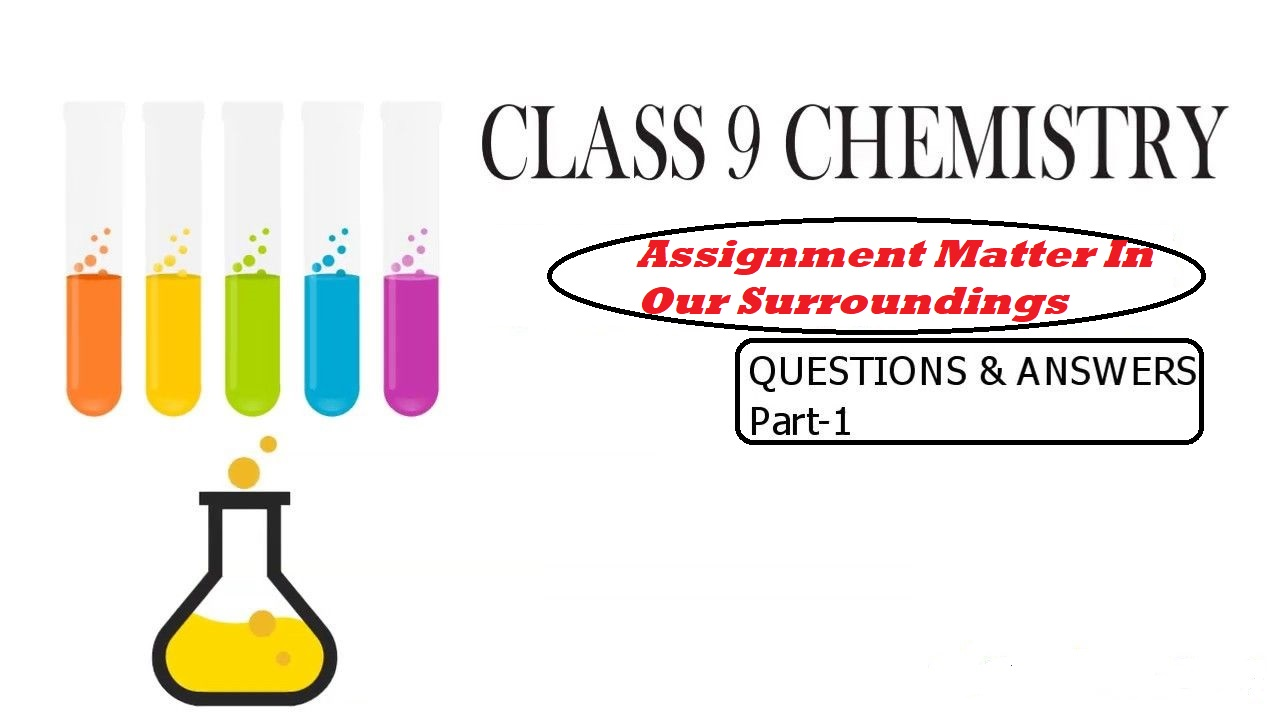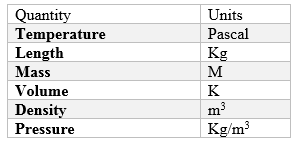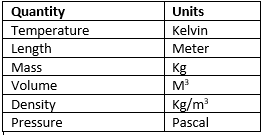# Assignment Matter In Our Surroundings### Assignment Matter In Our Surroundings

Assignment Matter In Our Surroundings

This post Assignment Matter In Our Surroundings is about various solved questions of the chapter Matter In Our Surroundings.

Question 1. Explain why?
i. A gas fills a vessel completely.
ii. Camphor disappears without leaving any residue.
iii. The temperature does not rise during the process of melting and boiling; through heat energy is constantly supplied.
iv. Water stored in an earthen vessel becomes cool.
v. An iron almirah is a solid at room temperature.

Solution1.

i). Because the molecules of the gas move freely so it occupies the whole space the vessel
ii) Camphor disappears after some time as its surface gains kinetic energy and gets directly converted into gas. The process of getting converted directly into a gas without getting converted into a liquid is called sublimation. So, we can say that camphor due to sublimation gets converted into gas and gets disappears after some time.

iii) The heat supplied is continuously used up in changing the state of the substance by overcoming the forces of attraction between the particles.
iv) Earthen pot has pores present on it which allow water, kept inside it to evaporate. This process takes energy from the water kept inside, as a result, the water becomes cool.
v) Iron has a high melting and boiling point. So it is solid at room temperature

Question 2. Explain why?
i. A gas fills a vessel completely.
ii. Camphor disappears without leaving any residue.
iii. The temperature does not rise during the process of melting and boiling; through heat energy is constantly supplied.
iv. Water stored in an earthen vessel becomes cool.
v. An iron almirah is a solid at room temperature.

Solution 2.

i) Sublimation
ii) Wax is in solid-state and it absorbs heat energy from the sun and starts melting
iii) Water molecules in the wet clothes absorb heat energy from Sun and evaporate to the atmosphere
iv) Water vapours formed from the ocean and other bodies. When it reaches the atmosphere, they get cooled because of low temperature and condense into water
v) Molecules are much closer in liquid as a comparison to the gas. Hence the Density of liquids is more than gases.

Question 3. Match the following:Solution 3.Question 4. Arrange the state in increasing order of the forces of attraction between the particles
a) Liquid
b) Gas
c) Solid

Solution 4Gas ->Liquid ->Solid

Question 5. On converting 25 0C, 38 0C, and 66 0C to kelvin scale, the correct sequence of temperature will be
(a) 298 K, 311 K, and 339 K
(b) 298 K, 300 K, and 338 K
(c) 273 K, 278 K, and 543 K
(d) 298 K, 310 K, and 338 K

Solution 5(a)

Question 6. Why does the temperature of a substance remain constant during its melting point or boiling point?

Solution 6The temperature of a substance remains constant at its melting and boiling points until all the substance melts or boils because the heat supplied is continuously used up in changing the state of the substance by overcoming the forces of attraction between the particles. This heat energy absorbed without showing any rise in temperature is given the name latent heat of fusion/latent heat of vaporization.

Question 7. What is condensation? How is the condensation of a gas carried out?

Solution 7The process due to which gas converts into a liquid state by giving out heat energy is called condensation. When a gas is compressed, it loses its heat energy thereby consequently decreasing the intermolecular distance between the molecules and increasing the intermolecular force of attraction between the molecules. Thus resulting in condensation of gas.

Question 8. Why do solids not diffuse?

Solution 8There is a strong intermolecular force of attraction between molecules and there is very little space for the molecules to move around.

Question 9. Convert the following Kelvin temperature to degrees Celsius.
a. 175 K
b. 295 K
c. 300 K
d. 225 K

Solution 9a. Degree Celsius = K – 273 = 175-273=-98 0C
b. 220C
c. 270C
d. -480C

Question 10. Convert the following Celsius temperature to Kelvin temperature.
a. 25 0C
b. -15 0C
c. 0 0C
d. 3 0C

Solution 10. a. Kelvin = 0C + 273 = 298k
b. 258K
c. 273K
d. 276K

Question 11.  What is the physical state of water at the following temperatures?
(a) 25 0C
(b) 0 0C
(c) 100 0C

Solution 11(a) 25 0C: liquid.
(b) 0 0C: solid or liquid (if melting process is on way).
(c) 100 0C: gas or liquid (if the boiling process is on way).

Question 12. Why do the gases exert more pressure on the walls of the container than the solids?

Solution 12Because gas molecules are in continuous random motion. They collide with each other and with the walls and apply pressure, but this is not observed in solid due to strong interaction

Question 13. A constant pressure tank of gas at 1.01 atm has propane in it at 150C when it is at 255 m3. What is its volume at 480C?

Solution 13This can be found using Charles law which states that at constant Volume is directly proportional to temperature, So
V1/T1=V2/T2
T1=15+273=288 K
T2=48+273=321
255/288 = V/321
V=284.2 m3

Question 14. Define the following terms:
a. Melting point
b. Freezing point
c. Boiling point

Solution 14. a. The temperature at which solid changes into a liquid.
b. The temperature at which liquid changes into solid.

c. The temperature at which liquid changes to vapours.

Question 15. Give three examples of crystalline and amorphous solids.

Solution 15Crystalline Solid – NaCl, CaF2, ZnS

Amorphous Solid – Rubber, Plastic, Glass.

Question 16. A rubber band can change its shape on stretching. Will you classify it as solid or not? Justify?

Solution 16Rubber band changes shape under force and regains the shape when the force is removed. So, it is classified as a solid.

Question 17. The liquid generally has a lower density as compared to solids, but ice floats on water. Find out, why?

Solution 17. Ice and water both are the same substance but ice has a greater volume than that of the same amount of water.
As density is inversely proportional to the volume, ice is less dense than water.
Objects having a density less than water float on the surface of the water.

#### This post Assignment Matter In Our Surroundings is about various solved questions of the chapter Matter In Our Surroundings.

Question 18.  What is dry ice?

Solution 18Solid CO2 is called dry ice because solid CO2 gets converted directly into the gaseous state without coming into the liquid state on decreasing pressure to one atmosphere.

Question 19. Give a reason to explain why it takes a longer time to dry wet clothes in humid weather?

Solution 19Rate of evaporation depends on the humidity present in the air. Humid air already has a large number of water vapours, so the rate of evaporation is slow.

Question 20. Why does a desert cooler cool better on a hot dry day?

Solution 20The humidity that is the number of water vapours in the air is less on a hot dry day. Lesser the humidity more is the rate of evaporation. Since the rate of evaporation is more on a hot dry day, a desert cooler cools better.

Assignment Matter In Our Surroundings will be updated with more questions.